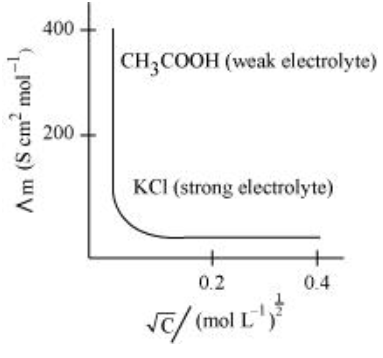# Define conductivity and molar conductivity for the solution of an electrolyte .

Question:

Define conductivity and molar conductivity for the solution of an electrolyte. Discuss their variation with concentration.

Solution:

Conductivity of a solution is defined as the conductance of a solution of 1 cm in length and area of cross-section 1 sq. cm. The inverse of resistivity is called conductivity or specific conductance. It is represented by the symbolκ. If ρ is resistivity, then we can write:

$\kappa=\frac{1}{\rho}$

The conductivity of a solution at any given concentration is the conductance (G) of one unit volume of solution kept between two platinum electrodes with the unit area of cross-section and at a distance of unit length.

i.e., $G=\kappa \frac{a}{l}=\kappa \cdot 1=\kappa$

(Since a = 1, l = 1)

Conductivity always decreases with a decrease in concentration, both for weak and strong electrolytes. This is because the number of ions per unit volume that carry the current in a solution decreases with a decrease in concentration.

Molar conductivity:

Molar conductivity of a solution at a given concentration is the conductance of volume V of a solution containing 1 mole of the electrolyte kept between two electrodes with the area of cross-section A and distance of unit length.

$\Lambda_{m}=\kappa \frac{A}{l}$

Now, l = 1 and A = V (volume containing 1 mole of the electrolyte).

$\therefore \Lambda_{m}=\kappa \mathrm{V}$

Molar conductivity increases with a decrease in concentration. This is because the total volume V of the solution containing one mole of the electrolyte increases on dilution.

The variation of $\Lambda_{m}$ with $\sqrt{\mathrm{c}}$ for strong and weak electrolytes is shown in the following plot: